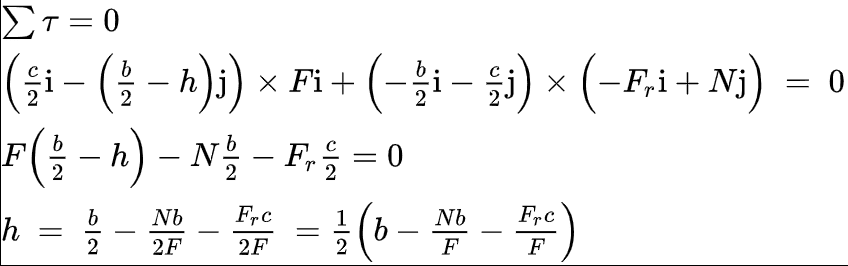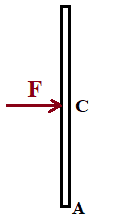# Rigid body sliding without overturning

Homework Statement:
A force F is applied to a box of weight P (rigid body). If u is the coefficient of friction, determine the minimum value for h that allows the body to slide without overturning backwards.
Relevant Equations:
L = r x p = r x mv
dL/dt = r x F
My attempt:

When the body is about to overturn backwards, the normal force and frictional force are applied on point A.
If I want to avoid overturning, torque must be 0. Then, I calculate torque with respect to A.
The value I get for h is negative, implying there is no minimum value for h.

What am I doing wrong?

Sorry if something is not clear, I am not a native english speaker.

#### Attachments

BvU
Homework Helper
Hello @gonza178,!

What am I doing wrong?
You have the idea you are doing something wrong. Why ?

Hint: consider a case that makes it very likely your result is indeed wrong. For example: ##b>>c## and ##h = 0## ...

You have the idea you are doing something wrong. Why ?

Hint: consider a case that makes it very likely your result is indeed wrong. For example: ##b>>c## and ##h = 0## ...

Hi!

I actually have considered the case you are telling me and thats why I think I am doing something wrong, my intuition tells me that if h is too small, the body is indeed likely to overturn. Also to be honest I asked my professor and the answer was "You are doing something wrong" but that is not really helpful...

If I do the same but I calculate torque with respect to the center of mass instead of A, I get a result that seems valid.But in the case that this is the correct answer, I am not quite understanding how a different axis of rotation affects the result.

etotheipi
Your original answer is correct, and you have made a mistake in your calculation of torque about the centre of mass. Notice that in the second term you have swapped ##b## and ##c## and it should actually be$$\left(-\frac{c}{2}\hat{i} -\frac{b}{2}\hat{j} \right) \times \left(-F_r \hat{i} + N \hat{j} \right)$$If you work that through, and use the fact that ##F_r = F## and ##N = P## for constant velocity, you will get the same as your original result!

BvU
Homework Helper
I actually have considered the case you are telling me and thats why I think I am doing something wrong, my intuition tells me that if h is too small, the body is indeed likely to overturn
Right ! Good intuition !

More questions of the same kind:
what if ##u=0##
You let N act at point A -- with good reason. Why ?
Where does the accelerating force have to act to only cause acceleration and no rotation ?

TSny
Homework Helper
Gold Member
I am not quite understanding how a different axis of rotation affects the result.

The sum of the torques on a body will depend on the choice of axis (if the net force on the body is nonzero). Consider the simple case of a meter stick in outer space that is initially at rest. A single force is then applied at the center of mass of the stick, as shown:The net torque about the center of mass is zero while the net torque about point A is nonzero. The stick does not begin to rotate even though the net torque about A is nonzero. Whenever the net force is nonzero (so the CM accelerates), you should use the net torque relative to the CM to determine if the object will begin to rotate.

Or, you can go to the reference frame moving with the stick. This will be a non-inertial frame. If you include the fictitious force acting at the center of mass, then you will get zero torque about both point C and point A. You could do this also for your block. Then you could take torques about the lower left corner.

Last edited:
•JD_PM and Lnewqban
BvU
Homework Helper
You mean ##h<0## ?????

etotheipi
You mean ##h<0## ?????

The OPs calculations assume zero acceleration of the centre of mass, in which case there can definitely be no rotation, so it sort of makes sense?

I think the problem does become a little more interesting if you do not constrain ##\vec{a} = \vec{0}##.Then you can continue from the last line of OPs working (with correction), $$h = \frac{1}{2}\left(b - \frac{bF_r}{F} - \frac{cN}{F} \right)$$and if limiting then ##F_r = \mu N \ (\neq F \text{, necessarily})##, and ##P = N##.

Last edited by a moderator:

Where does the accelerating force have to act to only cause acceleration and no rotation ?
Whenever the net force is nonzero (so the CM accelerates), you should use the net torque relative to the CM to determine if the object will begin to rotate.

Or, you can go to the reference frame moving with the stick. This will be a non-inertial frame. If you include the fictitious force acting at the center of mass, then you will get zero torque about both point C and point A.

This really helped me to understand what was happening.

jack action
Gold Member
The value I get for h is negative
No it's not. The only way the box can overturn about point A is if the force F is in the opposite direction, i.e. negative. In that case, h will be positive and your answer is good.

The way you have drawn your forces, the box would overturn about the opposite corner. And ##\frac{c}{2}\boldsymbol{i}## would become ##-\frac{c}{2}\boldsymbol{i}##.

•haruspex
etotheipi
No it's not. The only way the box can overturn about point A is if the force F is in the opposite direction, i.e. negative. In that case, h will be positive and your answer is good.

If we have the force applied as shown in the original diagram, shouldn't we then we have$$h = \frac{1}{2}\left(b - \frac{bF_r}{F} - \frac{cN}{F} \right)$$If ##F_r = \mu N## then$$h=\frac{1}{2}\left(b - \frac{b \mu N}{F} - \frac{cN}{F} \right) = \frac{1}{2}\left(b - \frac{P}{F} (b \mu + c) \right)$$with sufficiently small ##\frac{P}{F}## it would look like we can find some ##h>0## such that the horizontal force applied on the right results in some rotation (at least initially) about the bottom left corner. The box would also be accelerating horizontally.

Last edited by a moderator:
jack action
Gold Member
@etotheipi , I don't understand the use of the dimension b (even in the OP). I'm assuming it is related to b / 2, but what is at a distance b / 2?

etotheipi
@etotheipi , I don't understand the use of the dimension b (even in the OP). I'm assuming it is related to b / 2, but what is at a distance b / 2?

I think ##b## is the height of the box, and ##b/2## the vertical distance from the centre of mass to the ground.

jack action
Gold Member
OK, my mind was somewhere else.

$$h=\frac{1}{2}\left(b - \frac{b \mu N}{F} - \frac{cN}{F} \right) = \frac{1}{2}\left(b - \frac{P}{F} (b \mu + c) \right)$$
with sufficiently small ##\frac{P}{F}## it would look like we can find some ##h>0## such that the horizontal force applied on the right results in some rotation about the bottom left corner.
If we assume ##F >> P## then ##\frac{P}{F} = 0## and ##h = \frac{b}{2}##. This just means that ##F = ma##, basically ignoring the friction. Therefore, the box will not overturn.

The "largest" value will be ##\frac{P}{F} = -\frac{1}{\mu}##, which gives the case where there is no acceleration. (the negative sign assumes F is in the opposite direction) We then get the original ##h = \frac{c}{2\mu}##.

So ##h## will always range between ##\frac{b}{2}## and ##\frac{c}{2\mu}##, always a positive value, always assuming F is in the opposite direction, such that it overturns about point A.

haruspex
Homework Helper
Gold Member
2020 Award
@TSny has given you two ways to get the right answer in the accelerating case: take moments about the mass centre or, in a non-inertial frame, allow for the 'fictitious' force.
But the specific error in what you did was to equate zero torque to zero rotational acceleration of the body:
If I want to avoid overturning, torque must be 0. Then, I calculate torque with respect to A.
In the inertial frame of a fixed point on the ground, the linear acceleration of the block constitutes an angular acceleration around the axis. Therefore if the block is not tipping then the net torque about that axis is the moment of that acceleration about the axis.

etotheipi
I am not seeing what this has to do with @jack action's point. As he says in post #10, with the applied force being to the right, the potential turning point is also at the right hand end, not at the point A marked. This is why h came out negative.

OK but when the question said "slide without overturning backwards" I had assumed that meant tipping over in the opposite sense to the linear motion. I don't think it's possible for the box to overturn in this direction but it seems reasonable that the front end lifts up a bit if you accelerate it really quickly.

If we assume ##F >> P## then ##\frac{P}{F} = 0## and ##h = \frac{b}{2}##. This just means that ##F = ma##, basically ignoring the friction. Therefore, the box will not overturn.

Maybe I must look at this again, but that's not how I interpreted this. If ##\frac{P}{F} \rightarrow 0## then the range of ##h## required so that there is initial rotation of the box around its centre of mass, with the force in its position as shown on the diagram, will be ##h<\frac{b}{2}##.

•haruspex
haruspex
Homework Helper
Gold Member
2020 Award
OK but when the question said "slide without overturning backwards" I had assumed that meant tipping over in the opposite sense to the linear motion.
You are right - I misunderstood the question. Will delete my post.

haruspex